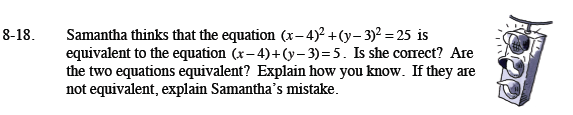Home > CCA2 > Chapter 8 > Lesson 8.1.1 > Problem8-18

8-18.Samantha thinks that the equation (x − 4)2 + (y − 3)2 = 25 is equivalent to the equation (x − 4) + (y − 3) = 5. Is she correct? Are the two equations equivalent? Explain how you know. If they are not equivalent, explain Samantha's mistake. Homework Help ✎Solve to find a point that makes the second equation true. Does it also make the first equation true?

Look at the graphs of each equation. Are they the same?